Bouquet

Gardener tying bouquet of flowers for 8 and none was left. Then he found that he could tying bouquet of 6 flowers and also none was left. How many have gardener flowers (minimum and maximum) if they had between 50 and 100 flowers?

Result

min =  72
max =  96

Solution:Leave us a comment of this math problem and its solution (i.e. if it is still somewhat unclear...):Be the first to comment!To solve this verbal math problem are needed these knowledge from mathematics:

Do you want to calculate least common multiple two or more numbers?

Next similar math problems:

1. Balls groups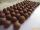Karel pulled the balls out of his pocket and divide it into the groups. He could divide them in four, six or seven, and no ball ever left. How little could be a ball?
2. Divisibility by 12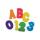Replace the letters A and B by digits so that the resulting number x is divisible by twelve /find all options/. x = 2A3B How many are the overall solutions?
3. Gears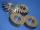The gearing fits wheel with 20 teeth to the wheel with 36 teeth. Before starting the machine is stained tooth smaller wheels in the designated space between the teeth of the larger wheels. How many times after starting the machine wheels turning that stain
4. Class 9AOn the final certificate have one quarter of the class 9A mark "C" of mathematics, seventh mark "C" from the Czech language and two students failed in chemistry. How many students attend class 9A?
5. Lcm simpleFind least common multiple of this two numbers: 140 175.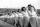Practitioners lined up in rectangle with row with four, five or six exercisers, one always missing to full rectangle. How many exercisers were on the field, if they have estimated not been more than 100?
7. Gearing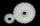Gearing consists of two wheels, one is 88 and second 56 teeth. How many times turns smaller wheel to fit the same teeth as in the beginning? How many times will turn biggest wheel?
8. Log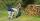Worker cut his thick log to 6 pieces for 30 min. How long he cut log to 12 pieces?
9. Counting numberWhat is the smallest counting number divisible by 2,5,7,8 and 15?
10. Lcm of three numbersWhat is the Lcm of 120 15 and 5
11. Plum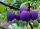On the platter are plum. How many were there if its have to be able to share equally among 8,10 and 12 children?
12. Trams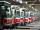Two trams started at the same time from the same place. One tram journey takes 30 minutes and the second 45 minutes to its final stop. How long will trams meet again?
13. School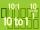Headteacher think whether the distribution of pupils in race in groups of 4,5,6,9 or 10. How many pupils must have at least school at possible options?
14. Tram lines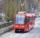Trams of five lines driven at intervals of 5,8,10,12 and 15 minutes. At 12 o'clock come out of the station at the same time. About how many hours again all meet? How many times have earch tram pass for this stop?
15. SickSick Marcel already taken six tablets, which was a quarter of the total number of pills in the pack. How many pills were in the pack?
16. Equation?
17. BiketrialKamil was biketrial. Before hill he set the forward gear with 42 teeth and the back with 35 teeth. After how many exercises (rotation) of the front wheel both wheels reach the same position?